深度学习笔记——Attention Model（注意力模型）学习总结

AM刚开始也确实是应用在图像领域里的，AM在图像处理领域取得了非常好的效果！于是，就有人开始研究怎么将AM模型引入到NLP领域。最有名的当属“Neural machine translation by jointly learning to align and translate”这篇论文了，这篇论文最早提出了Soft Attention Model，并将其应用到了机器翻译领域。后续NLP领域使用AM模型的文章一般都会引用这篇文章（目前引用量已经上千了！！！）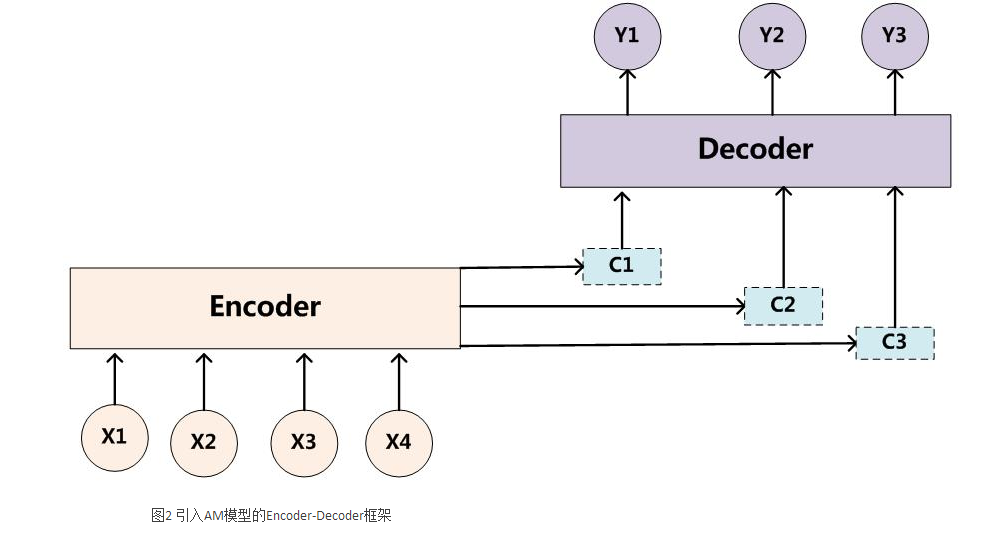Soft Attention Model：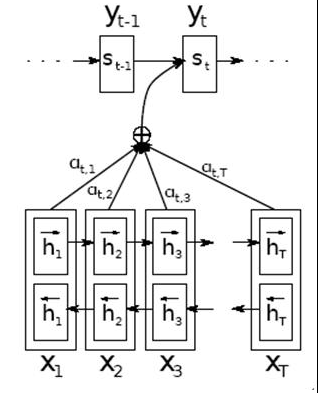Global Attention Model和Local Attention Model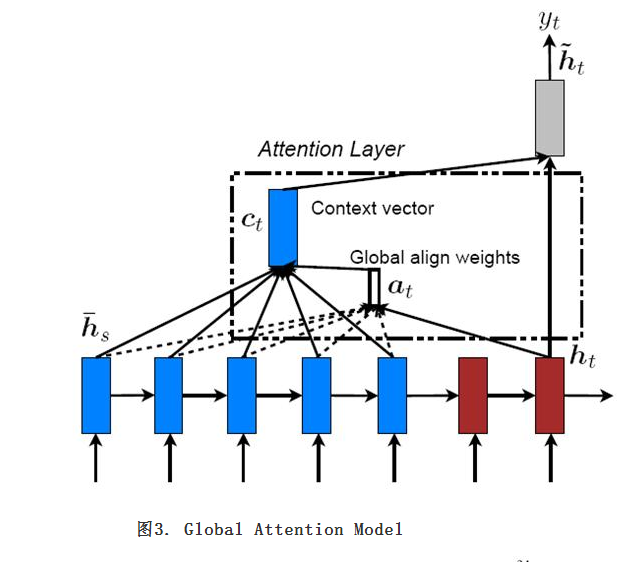Global AM其实就是soft AM，Decoder的过程中，每一个时间步的Context vector需要计算Encoder中每一个单词的注意力权重，然后加权得到。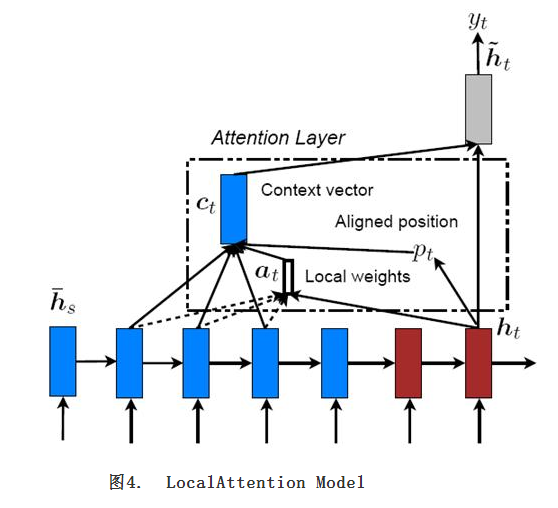Local AM则是首先找到一个对其位置，然后在对其位置左右一个窗口内来计算注意力权重，最终加权得到Context vector。这其实是Soft AM和Hard AM的一个混合折中。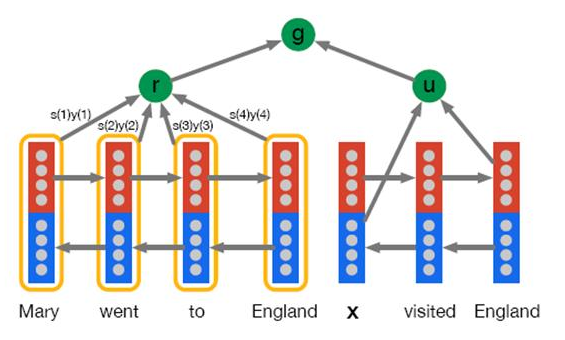Soft AM在逐步生成目标句子单词的时候，是由前向后逐步生成的，但是每个单词在求输入句子单词对齐模型时，并没有什么特殊要求。强制前向AM则增加了约束条件：要求在生成目标句子单词时，如果某个输入句子单词已经和输出单词对齐了，那么后面基本不太考虑再用它了，因为输入和输出都是逐步往前走的，所以看上去类似于强制对齐规则在往前走。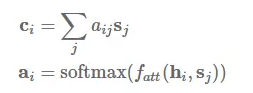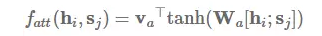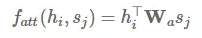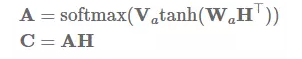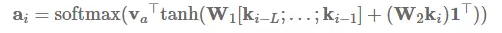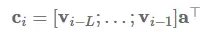from keras import backend as K
from keras.engine.topology import Layer
from keras import initializers, regularizers, constraints

class Attention_layer(Layer):
"""
Attention operation, with a context/query vector, for temporal data.
Follows the work of Yang et al. [https://www.cs.cmu.edu/~diyiy/docs/naacl16.pdf]
"Hierarchical Attention Networks for Document Classification"
by using a context vector to assist the attention
# Input shape
3D tensor with shape: (samples, steps, features).
# Output shape
2D tensor with shape: (samples, features).
:param kwargs:
Just put it on top of an RNN Layer (GRU/LSTM/SimpleRNN) with return_sequences=True.
The dimensions are inferred based on the output shape of the RNN.
Example:
"""

def __init__(self,
W_regularizer=None, b_regularizer=None,
W_constraint=None, b_constraint=None,
bias=True, **kwargs):

self.init = initializers.get('glorot_uniform')

self.W_regularizer = regularizers.get(W_regularizer)
self.b_regularizer = regularizers.get(b_regularizer)

self.W_constraint = constraints.get(W_constraint)
self.b_constraint = constraints.get(b_constraint)

self.bias = bias
super(Attention_layer, self).__init__(**kwargs)

def build(self, input_shape):
assert len(input_shape) == 3

initializer=self.init,
name='{}_W'.format(self.name),
regularizer=self.W_regularizer,
constraint=self.W_constraint)
if self.bias:
initializer='zero',
name='{}_b'.format(self.name),
regularizer=self.b_regularizer,
constraint=self.b_constraint)

super(Attention_layer, self).build(input_shape)

# do not pass the mask to the next layers
return None

uit = K.dot(x, self.W)

if self.bias:
uit += self.b

uit = K.tanh(uit)

a = K.exp(uit)

# apply mask after the exp. will be re-normalized next
# Cast the mask to floatX to avoid float64 upcasting in theano

# in some cases especially in the early stages of training the sum may be almost zero
# and this results in NaN's. A workaround is to add a very small positive number to the sum.
# a /= K.cast(K.sum(a, axis=1, keepdims=True), K.floatx())
a /= K.cast(K.sum(a, axis=1, keepdims=True) + K.epsilon(), K.floatx())
print a
# a = K.expand_dims(a)
print x
weighted_input = x * a
print weighted_input
return K.sum(weighted_input, axis=1)

def compute_output_shape(self, input_shape):
return (input_shape, input_shape[-1])